# Solution assignment 09 Quadratic equations (abc-formula)

### Assignment 9

For which value(s) of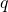does the equation: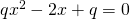have two coinciding solutions.

### Solution

In order to find out whether a quadratic equation has two different solutions, one (two coinciding) solution or no (real) solution at all we have to look at the discriminant. In this case: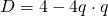The equation has two coinciding solutions if: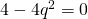or: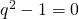We can calculate the values ofby solving the equation. This can be done in two different ways.
We can apply the special product: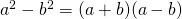In this case this means: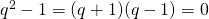and thus the solutions are: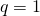or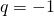We can also get the same result by solving: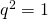but quite often the negative solution will be forgotten.

0